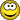# Confused why MUX implementation is not working:ClassicListThreaded2 messagesI am not sure if its that the logic is wrong or that I am making some syntax errors, but the file does not seem to load onto the hardware simulator and I am unsure what is wrong. Here is the implementation: And(a=a, b=b, out=AandB); And(sel=sel, b=b, out=BandSel); Not(in=b, out=NotB); Not(in=sel, out=NotSel); And(a=NotSel, b=NotB, out=NotSelNotB); And(a=a, b=NotSelNotB, out=out1); Or(a=BandSel, b=AandB, out=out2); Or(a=out2, b=out1, out=out); Any help would be greatly appreciated